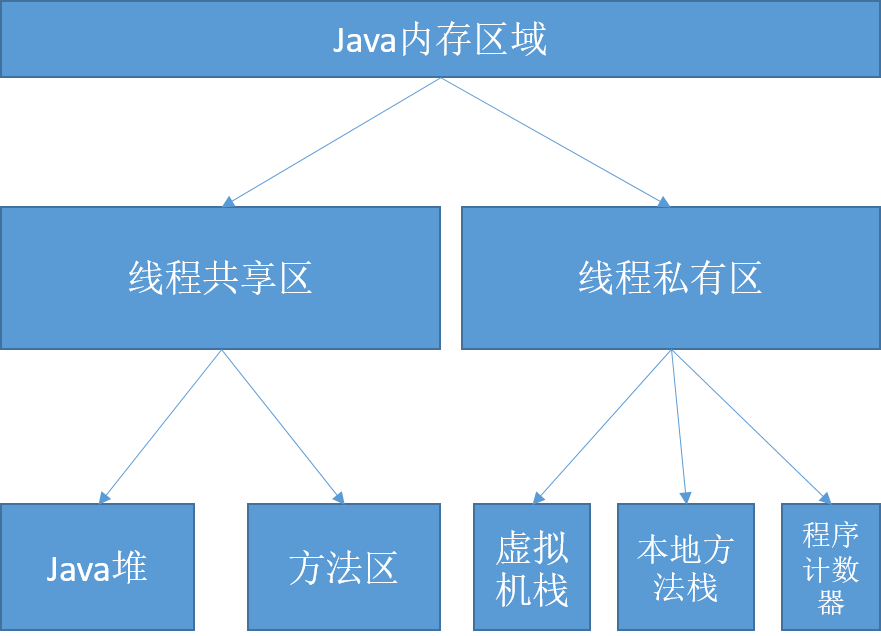# java中volatile关键字

Sep 12, 2016

## `volatile`到底有什么用

``````public class Main1 {
public static volatile int count = 0;

public static void main(String[] args) {

for (int i = 0; i < 10000; i++) {
count++;

}).start();
}
System.out.println(count);
}
}
``````

``````9972
``````

## jvm内存模型## `volatile`的真正作用

1. 线程1读取count的值，此时count的值为0
2. 线程2读取count的值，此时count的值为0
3. 线程1修改count的值为count+1=0+1=1，这个值会直接写到主内存，此时count的值为1
4. 线程2修改count的值为count+1=0+1，这个值再次写到主内存，count的值为1

## 什么时候使用`volatile`

``````public class Main {

static int count = 0;

public static void main(String[] args) {
// 线程1
do{}while (count<1);
System.out.println("exit when count = "+count);
}).start();
// 线程2
try {
count = 10;
System.out.println("change value of count to "+count);
} catch (InterruptedException e) {
e.printStackTrace();
}
}).start();
}
}
``````

1. 线程1启动，读取count的值为0，进入无限循环
2. 线程2启动，读取count的值为0，休眠1秒
3. 线程2休眠结束，修改count的值为10
4. 线程1的循环条件发现count=10>1，结束循环，打印出count的值
5. 程序结束

``````change value of count to 10
``````

``````static volatile int count = 0;
``````

``````change value of count to 10
exit when count = 10
``````

``````public static void main(String[] args) {
synchronized (count){
do{}while (count<1);
}
System.out.println("exit when count = "+count);
}).start();
try {
count = 10;
System.out.println("change value of count to "+count);
} catch (InterruptedException e) {
e.printStackTrace();
}
}).start();
}
``````

``````public static void main(String[] args) {
do{}while (count<1);
System.out.println("exit when count = "+count);
}).start();
try {
synchronized (count){
count = 10;
}
System.out.println("change value of count to "+count);
} catch (InterruptedException e) {
e.printStackTrace();
}
}).start();
}
``````

## 如何正确使用`volatile`

• 对变量的写操作不依赖于当前值
• 该变量没有包含在具有其他变量的不变式中

``````count = 10;
``````

``````count++;
``````

``````public class NumberRange {
private volatile int lower, upper;

public int getLower() { return lower; }
public int getUpper() { return upper; }

public void setLower(int value) {
if (value > upper)
throw new IllegalArgumentException(...);
lower = value;
}

public void setUpper(int value) {
if (value < lower)
throw new IllegalArgumentException(...);
upper = value;
}
}
``````

### 场景二：多线程初始化对象

``````public class Main {
public static void main(String[] args) {
ComplexObject obj = new ComplexObject();
// 线程1
do{
if(obj.field != null){
break;
}
}while (true);
System.out.println("exit when obj.field is not null ");
}).start();
// 线程2
try {
System.out.println("init obj");
obj.init();
} catch (InterruptedException e) {
e.printStackTrace();
}
}).start();
}

static class ComplexObject{
public volatile ComplexObject field = null;

public void init(){
field = new ComplexObject();
}

}
}
``````

### 场景四：不稳定对象

``````public class Person {
private volatile String firstName;
private volatile String lastName;
private volatile int age;

public String getFirstName() { return firstName; }
public String getLastName() { return lastName; }
public int getAge() { return age; }

public void setFirstName(String firstName) {
this.firstName = firstName;
}

public void setLastName(String lastName) {
this.lastName = lastName;
}

public void setAge(int age) {
this.age = age;
}
}
``````

#### 场景五：高性能读-写锁策略

``````public class Counter {
private volatile int value;

public int getValue() { return value; }

public synchronized int increment() {
return value++;
}
}
``````

## 总结

`volatile`的使用需要对jvm本身的一些机制特别理解才能正确使用，当然，既然涉及到jvm本身的机制，那么`volatile`的特性跟jvm的实现就有关系了，本文所有的代码和结论都是在jdk8下，使用`HotSpot`虚拟机得出的结果，不同的虚拟机可能会有不一样的实现结果，当然，只要符合按照jvm标准实现的虚拟机，上述的结论都是成立的。